# 采用SVM和神经网络的车牌识别（流程图及详细解释）

【原文：http://blog.csdn.net/raby_gyl/article/details/11659915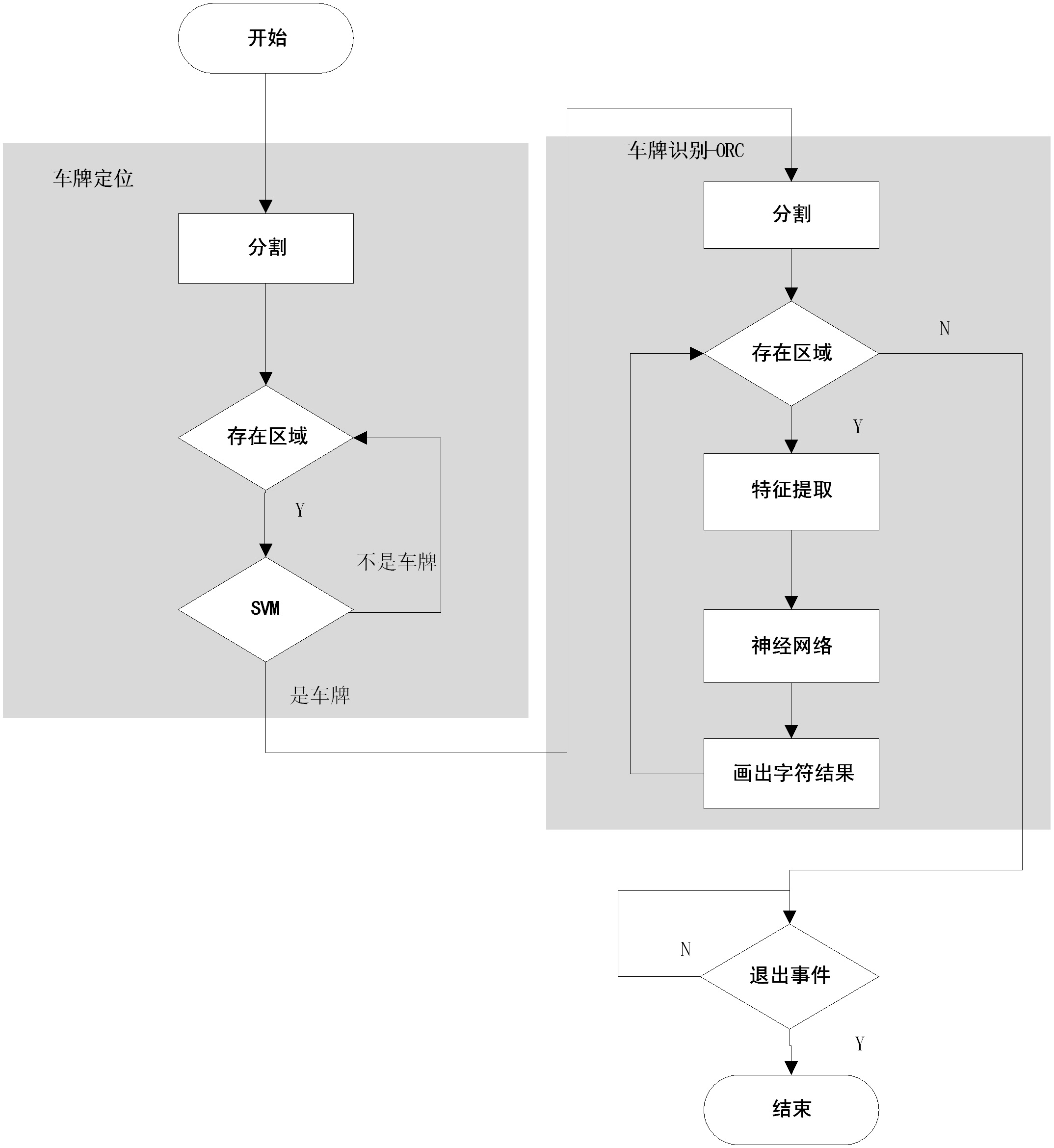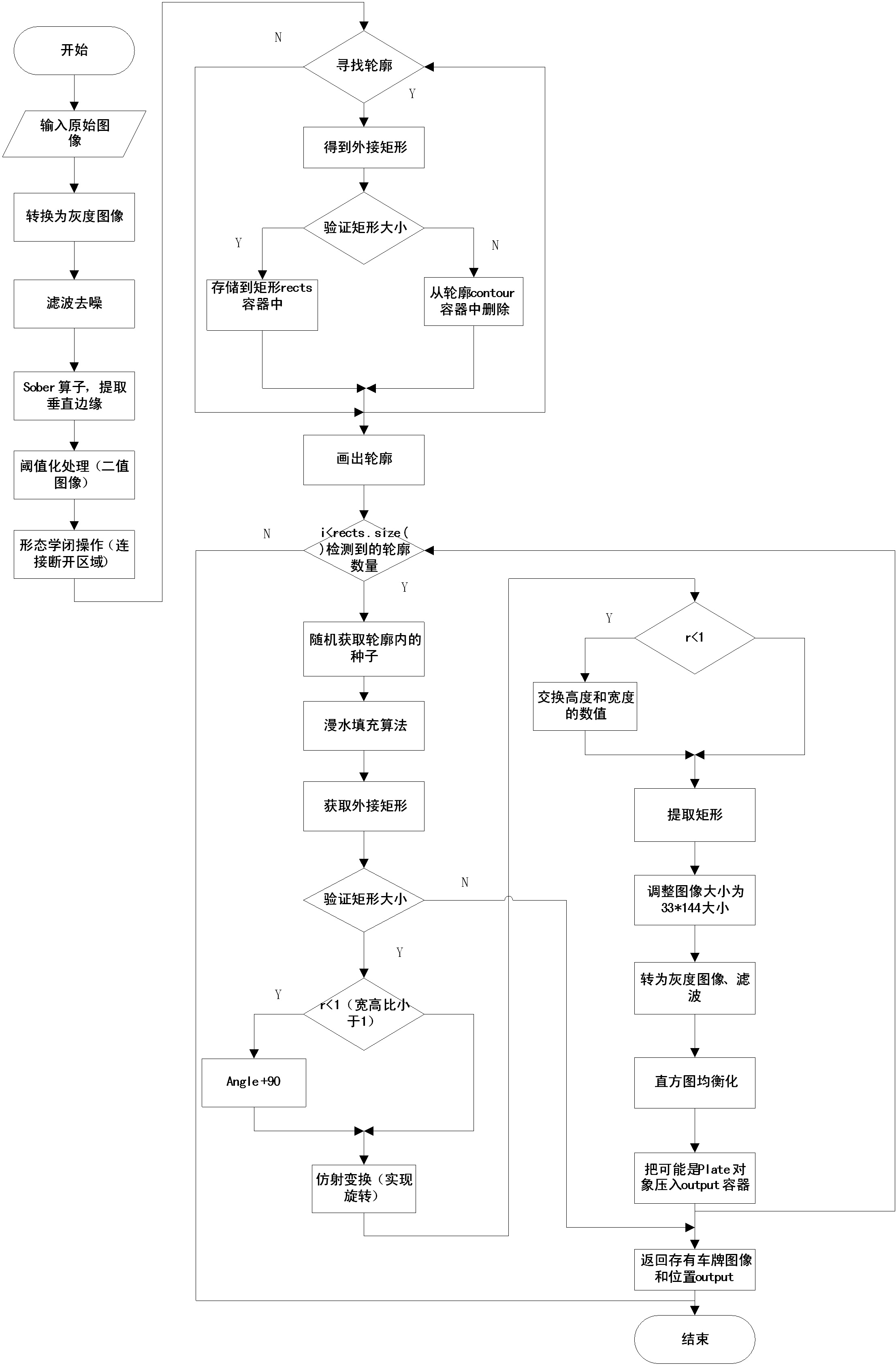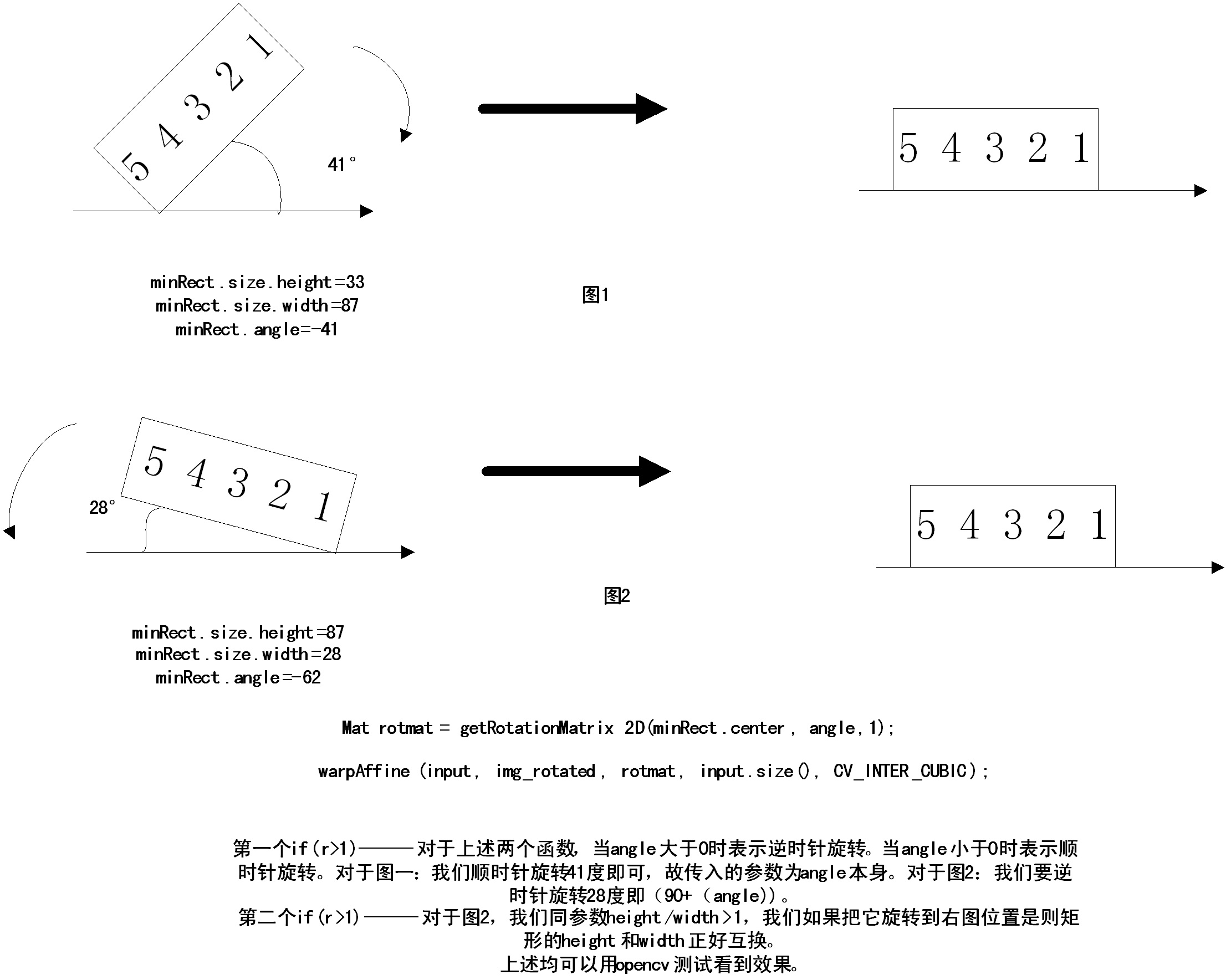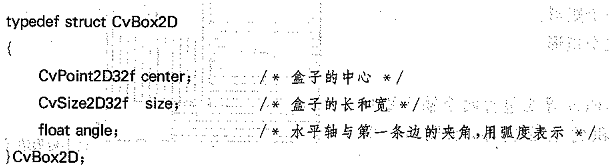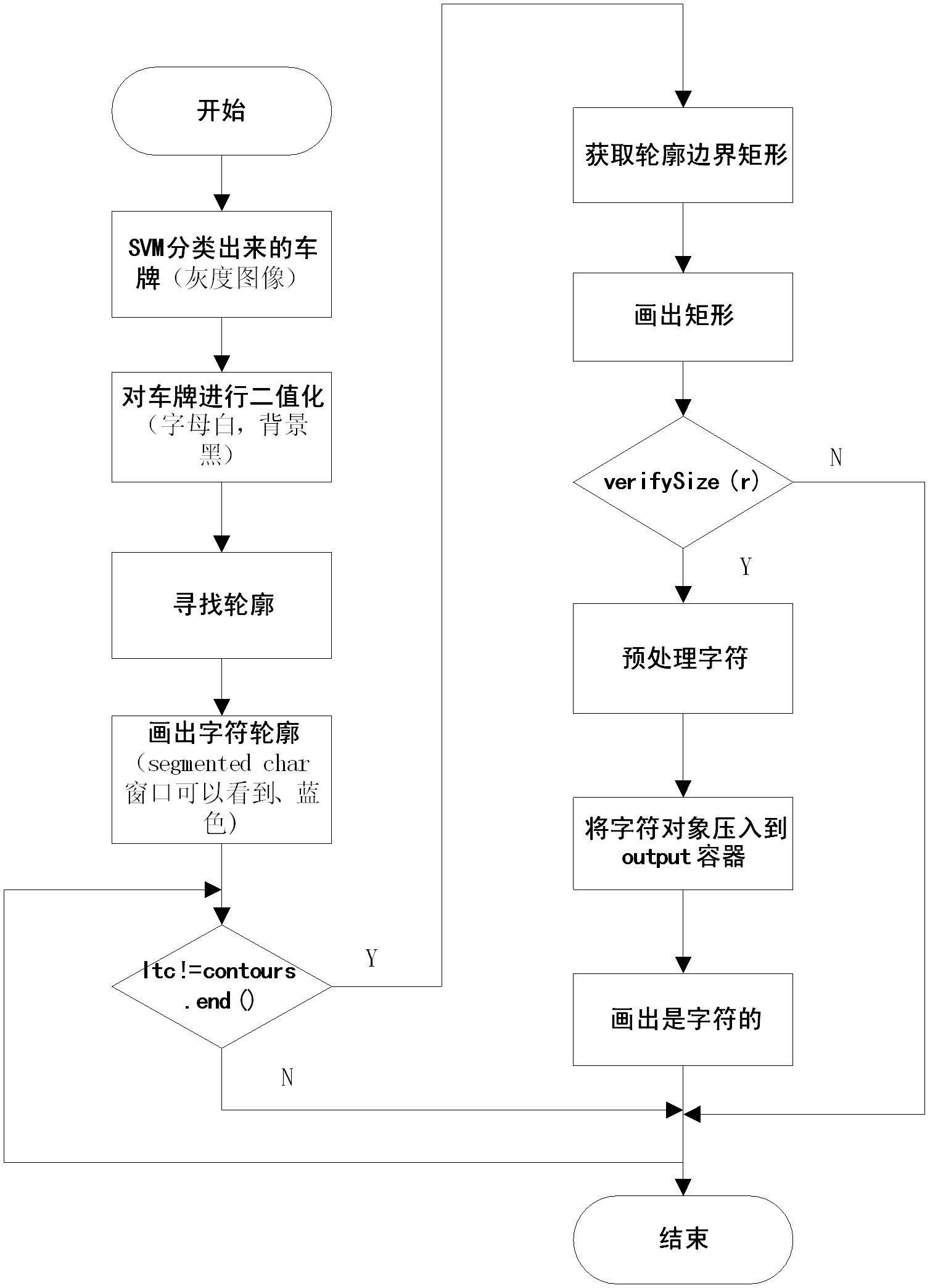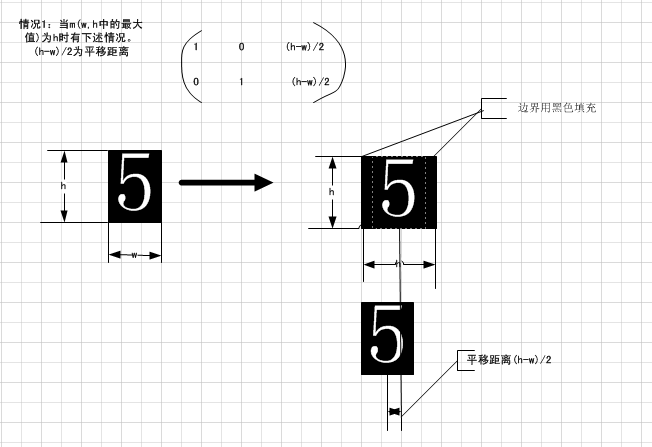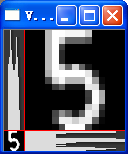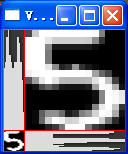《书中部分其他章节的翻译》http://blog.csdn.net/raby_gyl/article/details/17472271

<内容>

1、ANPR(自动车牌识别)

2、车牌检测

3、车牌识别

## ANPR介绍：

ANRP也就是众所周知的ALPR，或者AVI，或者CPR，是一种用在光学字符识别的监视方法和其他方法，例如分割和检测来读取车牌号。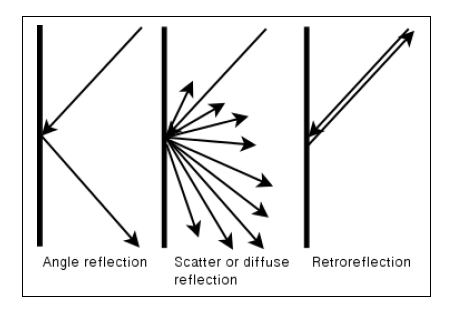角度反射             散射或者漫射          回复反射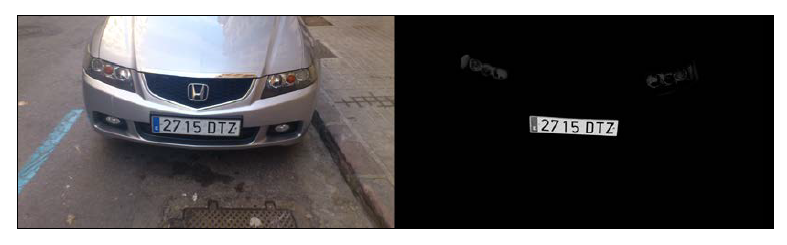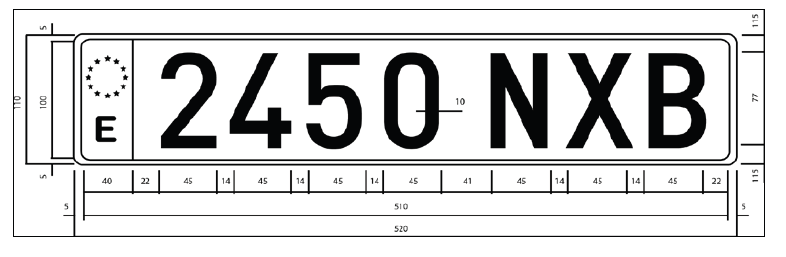## ANPR 算法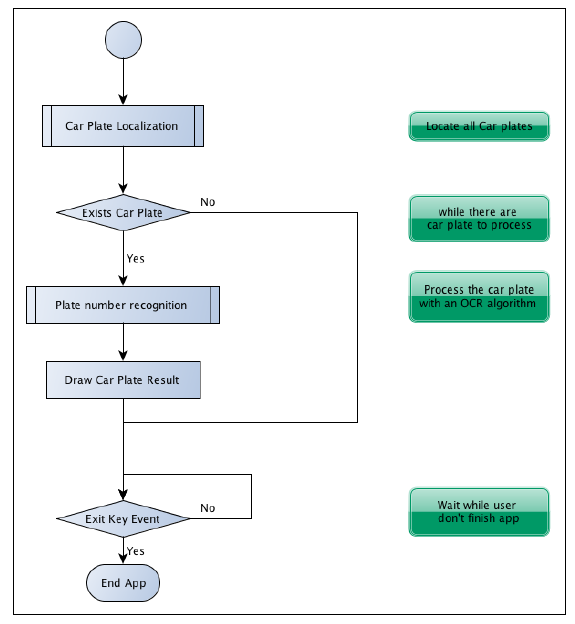1、分割。该步检测和移动图像中每个感兴趣的区域。

2、特征提取。该步提取每个块的一系列的特征。

3、分类。该步从车牌识别步骤或者把图像部分分为有车牌和无车牌的车牌检测的步骤，（上述两个步骤中）提取每个字符。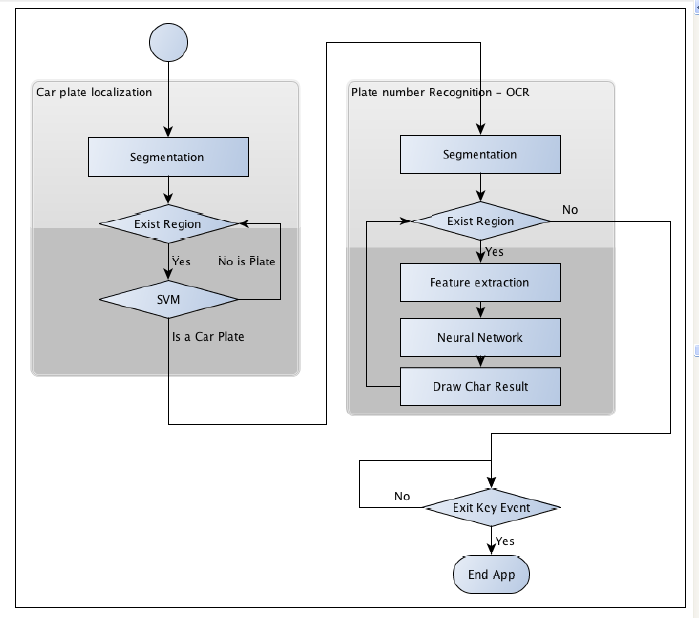1、怎么样训练一个模式识别系统？

2、怎么样来评估这样的一个系统？

## 车牌检测

1、Sobel滤波

2、阈值操作

3、闭操作

4、填充区域的掩膜

5、把可能检测到车牌标记为红色（特征图像）

6、SVM分类后，检测到的车牌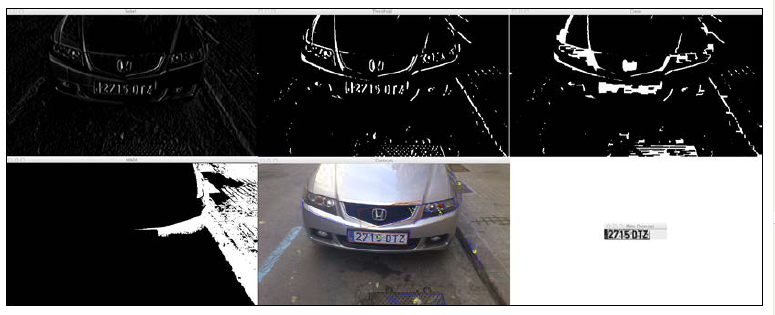## 分割

1. //convert image to gray
2. Mat img_gray;
3. cvtColor(input,img_gray,CV_BGR2GRAY);
4. blur(img_gray,img_gray,Size(5,5));

1. void Sobel(InputArray src,OutputArray dst,int ddepth,int xorder,int yoder,int ksize=3,double scale=1,double delta=0,int borderType=BORDER_DEFAULT)

1. //寻找垂直方向的线，车牌还很的垂直线密度
2. Mat img_sobel；
3. Sobel(img_gray,img_sobel,CV_8U,1,0,3,1,0);

Sobel滤波后，我们应用一个阈值滤波器来获得一个二值图像，阈值的通过otsu方法获得。Ostu算法需要一个8位图像作为输入，该方法自动的决定最佳的阈值。

1. Mat img_threshold;
2. threshold(img_sobel,img_threhold,0,255,CV_THRESH_OTSU+CV_THRESH_BINARY);

(小心：当CV_THRSH_OTST被定义，threshold函数会通过ostus算法返回最优阈值)

1. Mat element = getStructuringElement(MORPH_RECT, Size(17, 3));

1. morphologyEx(img_threshold, img_threshold, CV_MOP_CLOSE, element);

1. //Find contours of possibles plates
2. vector< vector< Point> > contours;
3. findContours(img_threshold,
4.             contours,           // a vector of contours
5.             CV_RETR_EXTERNAL,   // retrieve the external contours
6.             CV_CHAIN_APPROX_NONE); // all pixels of each contour

1. //Start to iterate to each contour found
2. vector<vector<Point> >::iterator itc= contours.begin();
3. vector<RotatedRect> rects;
4. //Remove patch that has  no inside limits of aspect ratio and area.
5. while (itc!=contours.end()) {
6. //Create bounding rect of object
7.   RotatedRect mr= minAreaRect(Mat(*itc));
8.   if( !verifySizes(mr)){
9.     itc= contours.erase(itc);
10.   }else{
11.   ++itc;
12.   rects.push_back(mr);
13.   }
14. }

1. bool DetectRegions::verifySizes(RotatedRect candidate ){
2.   float error=0.4;
3. //Spain car plate size: 52x11 aspect 4,7272
4.   const float aspect=4.7272;
5. //Set a min and max area. All other patches are discarded
6.   int min= 15*aspect*15; // minimum area
7.   int max= 125*aspect*125; // maximum area
8. //Get only patches that match to a respect ratio.
9.   float rmin= aspect-aspect*error;
10.   float rmax= aspect+aspect*error;
11.   int area= candidate.size.height * candidate.size.width;
12.   float r= (float)candidate.size.width / (float)candidate.size.height;
13.   if(r<1)
14. r= 1/r;
15.   if(( area < min || area > max ) || ( r < rmin || r > rmax )){
16.     return false;
17.   }else{
18.   return true;
19.   }
20. }

1. for(int i=0; i< rects.size(); i++){
2. //For better rect cropping for each possible box
3. //Make floodfill algorithm because the plate has white background
4. //And then we can retrieve more clearly the contour box
5. circle(result, rects[i].center, 3, Scalar(0,255,0), -1);
6. //get the min size between width and height
7. float minSize=(rects[i].size.width < rects[i].size.height)?rects[i].
8. size.width:rects[i].size.height;
9. minSize=minSize-minSize*0.5;
10. //initialize rand and get 5 points around center for floodfill
11. algorithm
12. srand ( time(NULL) );
13. //Initialize floodfill parameters and variables
15. mask.create(input.rows + 2, input.cols + 2, CV_8UC1);
17. int loDiff = 30;
18. int upDiff = 30;
19. int connectivity = 4;
20. int newMaskVal = 255;
21. int NumSeeds = 10;
22. Rect ccomp;
23. int flags = connectivity + (newMaskVal << 8 ) + CV_FLOODFILL_FIXED_
24. RANGE + CV_FLOODFILL_MASK_ONLY;
25. for(int j=0; j<NumSeeds; j++){
26.   Point seed;
27.   seed.x=rects[i].center.x+rand()%(int)minSize-(minSize/2);
28.   seed.y=rects[i].center.y+rand()%(int)minSize-(minSize/2);
29.   circle(result, seed, 1, Scalar(0,255,255), -1);
30.   int area = floodFill(input, mask, seed, Scalar(255,0,0), &ccomp,
31. Scalar(loDiff, loDiff, loDiff), Scalar(upDiff, upDiff, upDiff),
32. flags);
33.   }

1. int floodFill(InputOutputArray image, InputOutputArray mask, Point
2. seed, Scalar newVal, Rect* rect=0, Scalar loDiff=Scalar(), Scalar
3. upDiff=Scalar(), int flags=4 )

1、低位：包含连通的值，默认4连通，或者8连通。连通决定了像素的哪个邻居像素被考虑进来。

CV_FLOODFILL_FIXED_RANGE用来设置当前像素和种子像素之间的差异。

//检查新城的漫水填充掩码是不是一个正确的块。（因为使用车牌，车牌有边界，漫水填充不会超过车牌的边界，而对于其他区域（检查出来的矩形）漫水填充会占据很多区域，形成的矩形也很大，再进入verifySizes函数时，可能就会被丢弃，得到更可能是车牌的区域）

//得到所有的点为最小旋转矩形

1. //Check new floodfill mask match for a correct patch.
2. //Get all points detected for minimal rotated Rect
3. vector<Point> pointsInterest;
5. Mat_<uchar>::iterator end= mask.end<uchar>();
9.   RotatedRect minRect = minAreaRect(pointsInterest);
10.   if(verifySizes(minRect)){
11. …

1. //Get rotation matrix
2. float r= (float)minRect.size.width / (float)minRect.size.height;
3. float angle=minRect.angle;
4. if(r<1)
5.   angle=90+angle;
6.   Mat rotmat= getRotationMatrix2D(minRect.center, angle,1);

1. //Create and rotate image
2. Mat img_rotated;
3. warpAffine(input, img_rotated, rotmat, input.size(), CV_INTER_CUBIC);

1. //Crop image
2. Size rect_size=minRect.size;
3. if(r < 1)
4. swap(rect_size.width, rect_size.height);
5. Mat img_crop;
6. getRectSubPix(img_rotated, rect_size, minRect.center, img_crop);

1. Mat resultResized;
2. resultResized.create(33,144, CV_8UC3);
3. resize(img_crop, resultResized, resultResized.size(), 0, 0, INTER_
4. CUBIC);
5. //Equalize cropped image
6. Mat grayResult;
7. cvtColor(resultResized, grayResult, CV_BGR2GRAY);
8. blur(grayResult, grayResult, Size(3,3));
9. equalizeHist(grayResult, grayResult);

1. output.push_back(Plate(grayResult,minRect.boundingRect()));

## 分类

SVM创建一个或多个超平面，用来区分每类数据。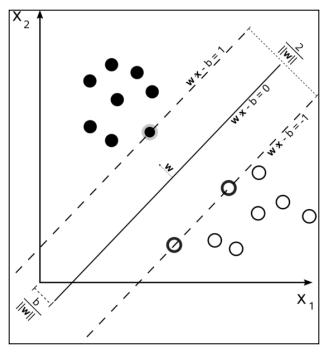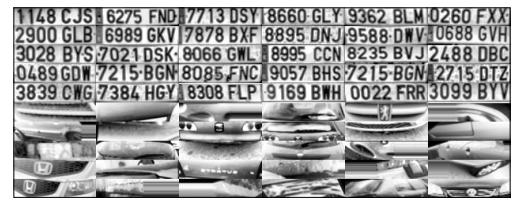opencv有个简单的方式来管理xml或者Json个数的数据文件，即FileStorage类。这个类使我们存储和读取opencv变量和结构体或者我们传统的变量。使用这个函数，我们嫩而过读取训练的数据矩阵和训练的类别，并且把他们保存在SVM_TrainingData 和SVM_Classes中：

1. FileStorage fs;
3. Mat SVM_TrainingData;
4. Mat SVM_Classes;
5. fs["TrainingData"] >> SVM_TrainingData;
6. fs["classes"] >> SVM_Classes;

1. //Set SVM params
2. CvSVMParams SVM_params;
3. SVM_params.kernel_type = CvSVM::LINEAR;

1. CvSVM svmClassifier(SVM_TrainingData, SVM_Classes, Mat(), Mat(), SVM_
2. params);

1. vector<Plate> plates;
2. for(int i=0; i< possible_regions.size(); i++)
3. {
4.   Mat img=possible_regions[i].plateImg;
5.   Mat p= img.reshape(1, 1);//convert img to 1 row m features
6.   p.convertTo(p, CV_32FC1);
7.   int response = (int)svmClassifier.predict( p );
8.   if(response==1)
9.     plates.push_back(possible_regions[i]);
10. }

## OCR分割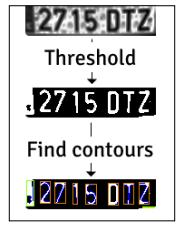1. Mat img_threshold;
2. threshold(input, img_threshold, 60, 255, CV_THRESH_BINARY_INV);
3. if(DEBUG)
4.   imshow("Threshold plate", img_threshold);
5. Mat img_contours;
6. img_threshold.copyTo(img_contours);
7. //Find contours of possibles characters
8. vector< vector< Point> > contours;
9. findContours(img_contours,
10.             contours,            // a vector of contours
11.             CV_RETR_EXTERNAL,    // retrieve the external contours
12.             CV_CHAIN_APPROX_NONE); // all pixels of each contour

1. bool OCR::verifySizes(Mat r)
2. {
3.   //Char sizes 45x77
4.   float aspect=45.0f/77.0f;
5.   float charAspect= (float)r.cols/(float)r.rows;
6.   float error=0.35;
7.   float minHeight=15;
8.   float maxHeight=28;
9.   //We have a different aspect ratio for number 1, and it can be
10.   //~0.2
11.   float minAspect=0.2;
12.   float maxAspect=aspect+aspect*error;
13.   //area of pixels
14.   float area=countNonZero(r);
15.   //bb area
16.   float bbArea=r.cols*r.rows;
17.   //% of pixel in area
18.   float percPixels=area/bbArea;
19.   if(percPixels < 0.8 && charAspect > minAspect && charAspect <
20.   maxAspect && r.rows >= minHeight && r.rows < maxHeight)
21.   return true;
22.   else
23.   return false;
24. }

## 特征提取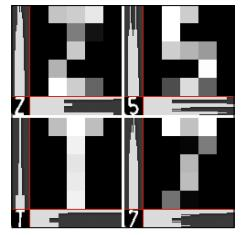1. Mat OCR::ProjectedHistogram(Mat img, int t)
2. {
3.   int sz=(t)?img.rows:img.cols;
4.   Mat mhist=Mat::zeros(1,sz,CV_32F);
5.   for(int j=0; j<sz; j++){
6.     Mat data=(t)?img.row(j):img.col(j);
7.     mhist.at<float>(j)=countNonZero(data);
8.   }
9. //Normalize histogram
10. double min, max;
11. minMaxLoc(mhist, &min, &max);
12. if(max>0)
13. mhist.convertTo(mhist,-1 , 1.0f/max, 0);
14.   return mhist;
15. }

1. Mat OCR::features(Mat in, int sizeData)
2. {
3. //Histogram features
4.   Mat vhist=ProjectedHistogram(in,VERTICAL);
5.   Mat hhist=ProjectedHistogram(in,HORIZONTAL);
6. //Low data feature
7.   Mat lowData;
8.   resize(in, lowData, Size(sizeData, sizeData) );
9.   int numCols=vhist.cols + hhist.cols + lowData.cols *
10.   lowData.cols;
11.   Mat out=Mat::zeros(1,numCols,CV_32F);
12.   //Assign values to feature
13.   int j=0;
14.   for(int i=0; i<vhist.cols; i++)
15.   {
16.     out.at<float>(j)=vhist.at<float>(i);
17.     j++;
18.   }
19.   for(int i=0; i<hhist.cols; i++)
20.   {
21.   out.at<float>(j)=hhist.at<float>(i);
22.   j++;
23.   }
24.   for(int x=0; x<lowData.cols; x++)
25.   {
26.     for(int y=0; y<lowData.rows; y++)
27.     {
28.       out.at<float>(j)=(float)lowData.at<unsigned char>(x,y);
29.       j++;
30.     }
31.   }
32.   return out;
33. }

## OCR分类

MLP神经网络有一个输入层，输出层和一个或多个隐层。每一层有一个活多个神经元连接着前向和后向层。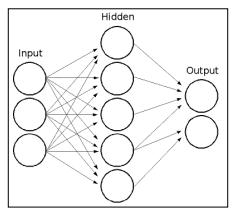MLP中所有的神经元，都相似，每个神经元有几个输出（前向连接神经元）和几个输出连接着相同的值（后向连接神经元）。每个神经元用带有权重的输入的和加上一个偏移量再经过一个选择的激活函数转换后得到输出结果。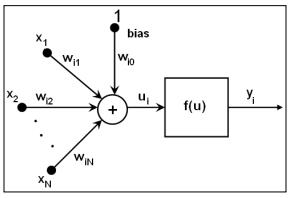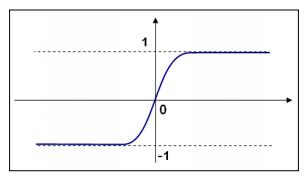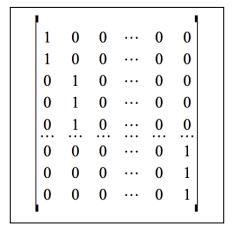Opencv为ANN定义了一个CvANN_MLP类。通过定义的层的数目和原子数，激活函数和alpha和beta参数用创建函数来初始化类。

1. void OCR::train(Mat TrainData, Mat classes, int nlayers)
2. {
3.   Mat layerSizes(1,3,CV_32SC1);
4.   layerSizes.at<int>(0)= TrainData.cols;
5.   layerSizes.at<int>(1)= nlayers;
6.   layerSizes.at<int>(2)= numCharacters;
7.   ann.create(layerSizes, CvANN_MLP::SIGMOID_SYM, 1, 1); //ann is
8.   global class variable
9.   //Prepare trainClasses
10.   //Create a mat with n trained data by m classes
11.   Mat trainClasses;
12.   trainClasses.create( TrainData.rows, numCharacters, CV_32FC1 );
13.   for( int i = 0; i <  trainClasses.rows; i++ )
14.   {
15.     for( int k = 0; k < trainClasses.cols; k++ )
16.     {
17.     //If class of data i is same than a k class
18.     if( k == classes.at<int>(i) )
19.     trainClasses.at<float>(i,k) = 1;
20.     else
21.     trainClasses.at<float>(i,k) = 0;
22.     }
23.   }
24.   Mat weights( 1, TrainData.rows, CV_32FC1, Scalar::all(1) );
25.   //Learn classifier
26.   ann.train( TrainData, trainClasses, weights );
27.   trained=true;
28. }

1. int OCR::classify(Mat f)
2. {
3.   int result=-1;
4.   Mat output(1, numCharacters, CV_32FC1);
5.   ann.predict(f, output);
6.   Point maxLoc;
7.   double maxVal;
8.   minMaxLoc(output, 0, &maxVal, 0, &maxLoc);
9.   //We need to know where in output is the max val, the x (cols) is
10.   //the class.
11.   return maxLoc.x;
12. }

CvANN_MLP类使用predict函数来为一个特征矢量分类。不想SVM的分类函数，ANN的predict函数返回的是一个矢量，其大小和类的数目相同，带有属于每一个类的输入特征的几率。（计算矢量中的每一个值，相当于该矢量属于概率的概率，大题可以这样认为）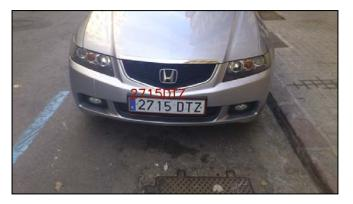2. rectangle(input_image, plate.position, Scalar(0,0,200));
3. putText(input_image, licensePlate, Point(plate.position.x, plate.
4. position.y), CV_FONT_HERSHEY_SIMPLEX, 1, Scalar(0,0,200),2);

## 评估

1. Mat classes;
2. Mat trainingData;
3. //Read file storage.
4. FileStorage fs;
6. fs[data] >> trainingData;
7. fs["classes"] >> classes;

1. float test(Mat samples, Mat classes)
2. {
3.   float errors=0;
4.   for(int i=0; i<samples.rows; i++)
5.   {
6.     int result= ocr.classify(samples.row(i));
7.     if(result!= classes.at<int>(i))
8.     errors++;
9.   }
10.   return errors/samples.rows;
11. }

1. #!/bin/bash
2. echo "#ITS \t 5 \t 10 \t 15 \t 20" > data.txt
3. folder=$(pwd) 4. for numNeurons in 10 20 30 40 50 60 70 80 90 100 120 150 200 500 5. do 6. s5=0; 7. s10=0; 8. s15=0; 9. s20=0; 10. for j in {1..100} 11. do 12. echo$numNeurons $j 13. a=$($folder/build/evalOCR$numNeurons TrainingDataF5)
14.     s5=$(echo "scale=4;$s5+$a" | bc -q 2>/dev/null) 15. a=$($folder/build/evalOCR$numNeurons TrainingDataF10)
16.     s10=$(echo "scale=4;$s10+$a" | bc -q 2>/dev/null) 17. a=$($folder/build/evalOCR$numNeurons TrainingDataF15)
18.     s15=$(echo "scale=4;$s15+$a" | bc -q 2>/dev/null) 19. a=$($folder/build/evalOCR$numNeurons TrainingDataF20)
20.     s20=$(echo "scale=4;$s20+$a" | bc -q 2>/dev/null) 21. done 22. echo "$i \t $s5 \t$s10 \t $s15 \t$s20"
23.   echo "$i \t$s5 \t $s10 \t$s15 \t \$s20" >> data.txt
24. done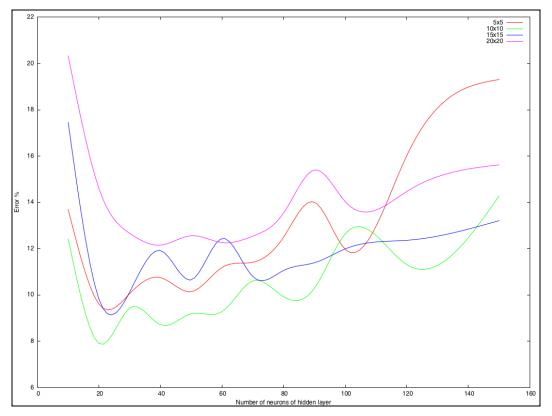## 总结